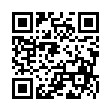# North Coast Synthesis Ltd.

## F+2+4+♯2Choose audio sample set:
MSK 007 Leapfrog VCF (patch; see in online store)
MSK 009 Coiler VCF (patch; see in online store)

Equivalent chord symbols: F+4+9+♯2, E♯+2+4+♯2, E♯+4+9+♯2, F+2+11+♯2, E♯+2+11+♯2.

Notes in this chord: F, G, G♯, A, B♭, C. Integer notation: {0, 5, 7, 8, 9, 10}.

Nearby chords (one less note): F+2+4, F+2+♯2, F+4+♯2, Fm+2+4, F4+2+♯2, Gm4+2+♯1.

Nearby chords (one more note): F11+♯2, FM11+♯2, Gm11+♯1, E♭13-1+♯4, F+2+4+♯1+♯2, F+2+4+♯2+♯4.

Parallel chords (same structure, different root): C+2+4+♯2, D+2+4+♯2, E+2+4+♯2, G+2+4+♯2, A+2+4+♯2, B+2+4+♯2, C♭+2+4+♯2, D♭+2+4+♯2, E♭+2+4+♯2, F♭+2+4+♯2, G♭+2+4+♯2, A♭+2+4+♯2, B♭+2+4+♯2, C♯+2+4+♯2, D♯+2+4+♯2, E♯+2+4+♯2, F♯+2+4+♯2, G♯+2+4+♯2, A♯+2+4+♯2, B♯+2+4+♯2.

Experimental fretting charts for guitar standard EADGBE tuning (change tuning or instrument):